# Thermodynamics numericl 2016

2 de Mar de 2018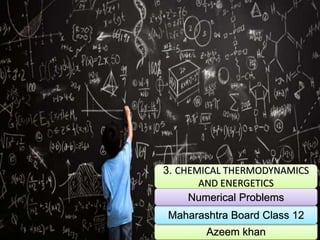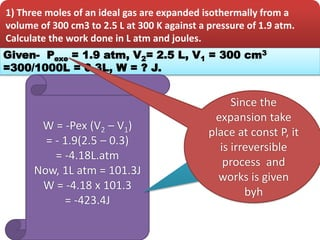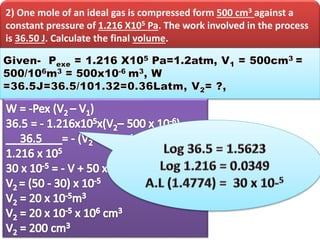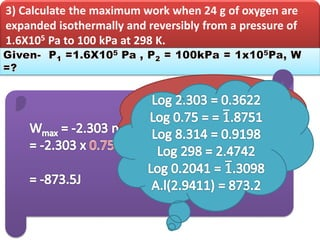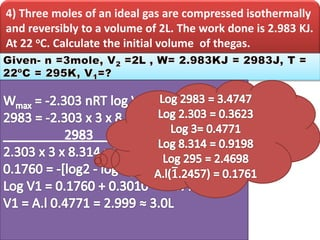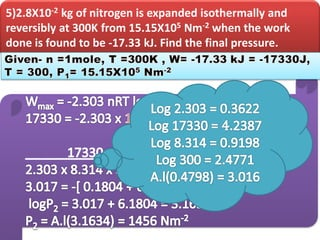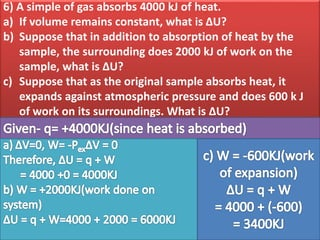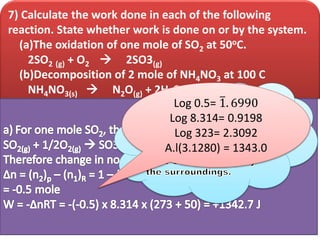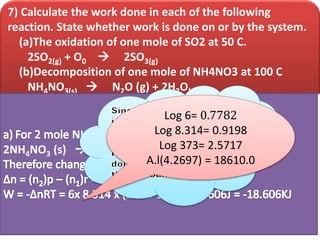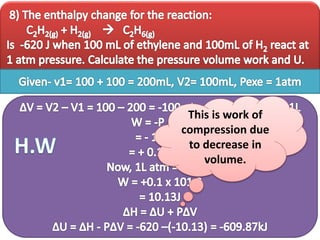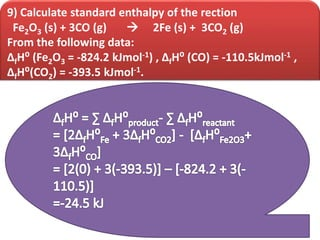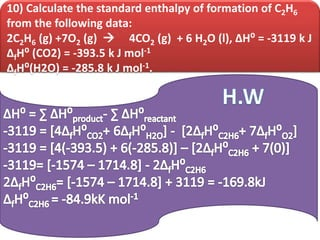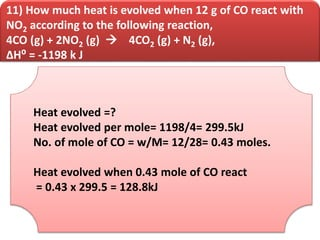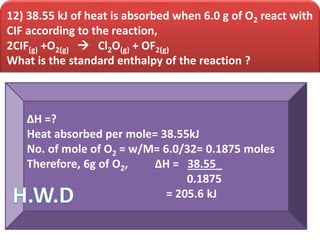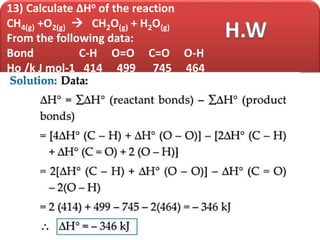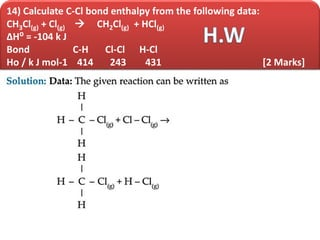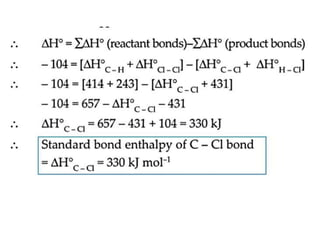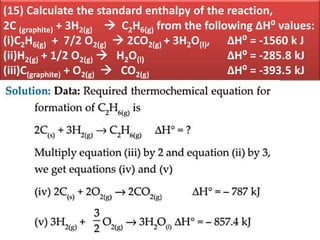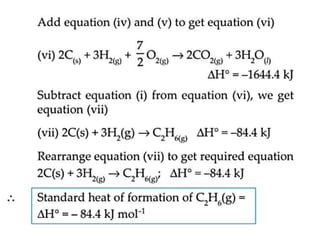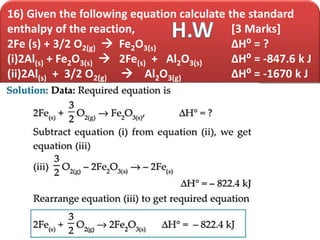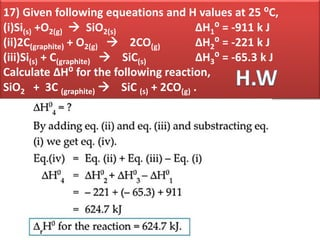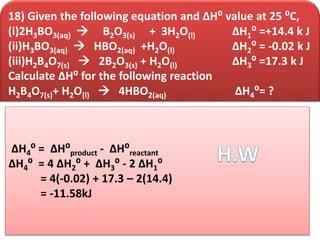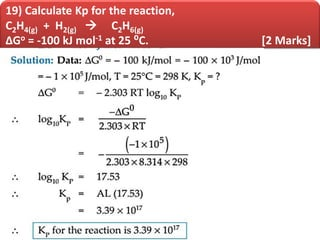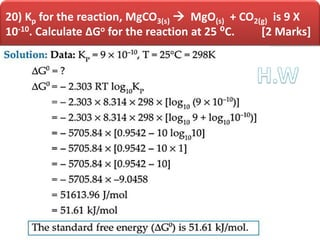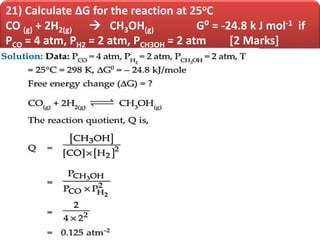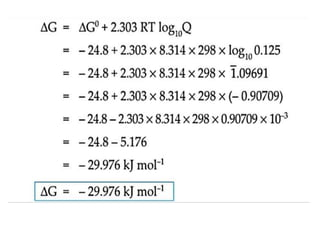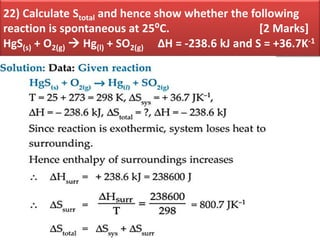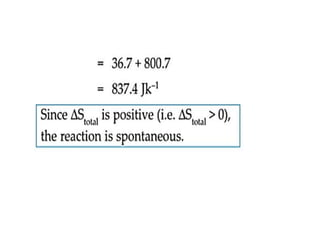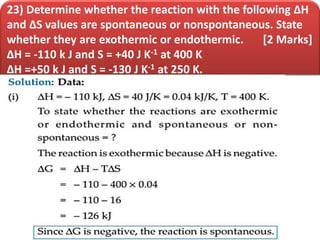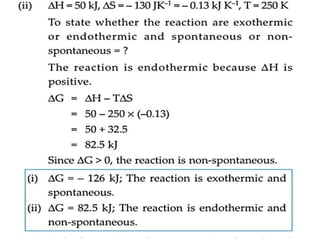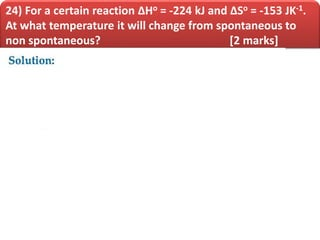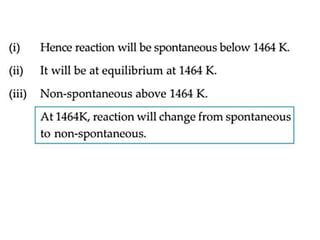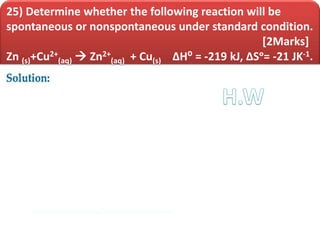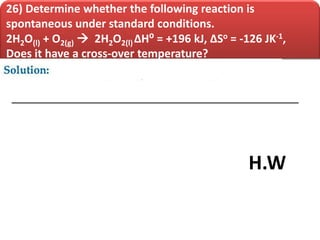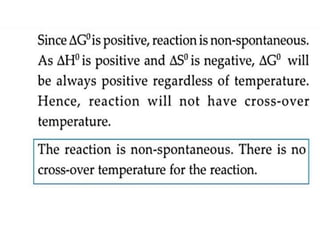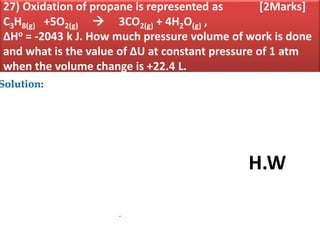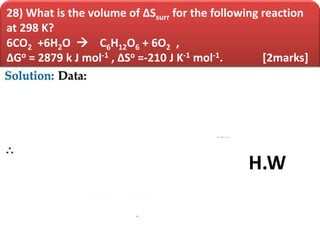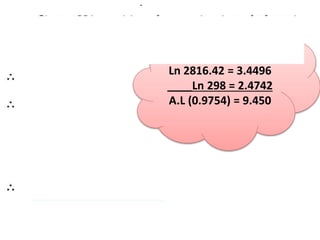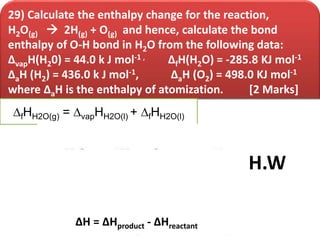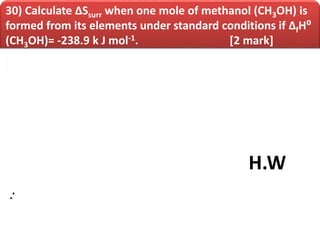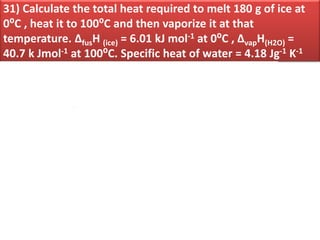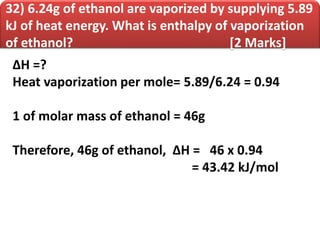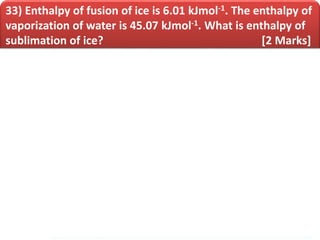1 de 45

### Thermodynamics numericl 2016

• 1. 3. CHEMICAL THERMODYNAMICS AND ENERGETICS Azeem khan Maharashtra Board Class 12 Numerical Problems
• 2. 1) Three moles of an ideal gas are expanded isothermally from a volume of 300 cm3 to 2.5 L at 300 K against a pressure of 1.9 atm. Calculate the work done in L atm and joules. Given- Pexe = 1.9 atm, V2= 2.5 L, V1 = 300 cm3 =300/1000L = 0.3L, W = ? J. W = -Pex (V2 – V1) = - 1.9(2.5 – 0.3) = -4.18L.atm Now, 1L atm = 101.3J W = -4.18 x 101.3 = -423.4J Log 4.18 = 0.6212 Log 101.3 = 2.0056 = 5.6268 A.l(5.6268) = 423.5 Since the expansion take place at const P, it is irreversible process and works is given byh
• 3. 2) One mole of an ideal gas is compressed form 500 cm3 against a constant pressure of 1.216 X105 Pa. The work involved in the process is 36.50 J. Calculate the final volume.
• 4. 3) Calculate the maximum work when 24 g of oxygen are expanded isothermally and reversibly from a pressure of 1.6X105 Pa to 100 kPa at 298 K. n o2 = Wo2 = 24 = 0.75 mol Mo2 32 Log 1.6 = 0.2041
• 5. 4) Three moles of an ideal gas are compressed isothermally and reversibly to a volume of 2L. The work done is 2.983 KJ. At 22 oC. Calculate the initial volume of thegas.
• 6. 5)2.8X10-2 kg of nitrogen is expanded isothermally and reversibly at 300K from 15.15X105 Nm-2 when the work done is found to be -17.33 kJ. Find the final pressure.
• 7. 6) A simple of gas absorbs 4000 kJ of heat. a) If volume remains constant, what is ∆U? b) Suppose that in addition to absorption of heat by the sample, the surrounding does 2000 kJ of work on the sample, what is ∆U? c) Suppose that as the original sample absorbs heat, it expands against atmospheric pressure and does 600 k J of work on its surroundings. What is ∆U?
• 8. 7) Calculate the work done in each of the following reaction. State whether work is done on or by the system. (a)The oxidation of one mole of SO2 at 50oC. 2SO2 (g) + O2  2SO3(g) (b)Decomposition of 2 mole of NH4NO3 at 100 C NH4NO3(s)  N2O(g) + 2H2O(g) Log 0.5= 1. 6990 Log 8.314= 0.9198 Log 323= 2.3092 A.l(3.1280) = 1343.0
• 9. 7) Calculate the work done in each of the following reaction. State whether work is done on or by the system. (a)The oxidation of one mole of SO2 at 50 C. 2SO2(g) + O0  2SO3(g) (b)Decomposition of one mole of NH4NO3 at 100 C NH4NO3(s)  N2O (g) + 2H2O(g) NH4NO3 2NH4NO3 (s)  2N2O (g) + 4H2O (g) Log 6= 0.7782 Log 8.314= 0.9198 Log 373= 2.5717 A.l(4.2697) = 18610.0
• 10. This is work of compression due to decrease in volume.
• 11. 9) Calculate standard enthalpy of the rection Fe2O3 (s) + 3CO (g)  2Fe (s) + 3CO2 (g) From the following data: ∆fH⁰ (Fe2O3 = -824.2 kJmol-1) , ∆fH⁰ (CO) = -110.5kJmol-1 , ∆fH⁰(CO2) = -393.5 kJmol-1.
• 12. 10) Calculate the standard enthalpy of formation of C2H6 from the following data: 2C2H6 (g) +7O2 (g)  4CO2 (g) + 6 H2O (l), ∆H⁰ = -3119 k J ∆fH⁰ (CO2) = -393.5 k J mol-1 ∆fH⁰(H2O) = -285.8 k J mol-1.
• 13. 11) How much heat is evolved when 12 g of CO react with NO2 according to the following reaction, 4CO (g) + 2NO2 (g)  4CO2 (g) + N2 (g), ∆H⁰ = -1198 k J Heat evolved =? Heat evolved per mole= 1198/4= 299.5kJ No. of mole of CO = w/M= 12/28= 0.43 moles. Heat evolved when 0.43 mole of CO react = 0.43 x 299.5 = 128.8kJ
• 14. 12) 38.55 kJ of heat is absorbed when 6.0 g of O2 react with CIF according to the reaction, 2CIF(g) +O2(g)  Cl2O(g) + OF2(g) What is the standard enthalpy of the reaction ? ∆H =? Heat absorbed per mole= 38.55kJ No. of mole of O2 = w/M= 6.0/32= 0.1875 moles Therefore, 6g of O2, ∆H = 38.55_ 0.1875 = 205.6 kJ
• 15. 13) Calculate ∆Ho of the reaction CH4(g) +O2(g)  CH2O(g) + H2O(g) From the following data: Bond C-H O=O C=O O-H Ho /k J mol-1 414 499 745 464
• 16. 14) Calculate C-Cl bond enthalpy from the following data: CH3Cl(g) + Cl(g)  CH2Cl(g) + HCl(g) ∆H⁰ = -104 k J Bond C-H Cl-Cl H-Cl Ho / k J mol-1 414 243 431 [2 Marks]
• 18. (15) Calculate the standard enthalpy of the reaction, 2C (graphite) + 3H2(g)  C2H6(g) from the following ∆H⁰ values: (i)C2H6(g) + 7/2 O2(g)  2CO2(g) + 3H2O(l), ∆H⁰ = -1560 k J (ii)H2(g) + 1/2 O2(g)  H2O(l) ∆H⁰ = -285.8 kJ (iii)C(graphite) + O2(g)  CO2(g) ∆H⁰ = -393.5 kJ
• 20. 16) Given the following equation calculate the standard enthalpy of the reaction, [3 Marks] 2Fe (s) + 3/2 O2(g)  Fe2O3(s) ∆H⁰ = ? (i)2Al(s) + Fe2O3(s)  2Fe(s) + Al2O3(s) ∆H⁰ = -847.6 k J (ii)2Al(s) + 3/2 O2(g)  Al2O3(g) ∆H⁰ = -1670 k J
• 21. 17) Given following equeations and H values at 25 ⁰C, (i)Si(s) +O2(g)  SiO2(s) ∆H1⁰ = -911 k J (ii)2C(graphite) + O2(g)  2CO(g) ∆H2⁰ = -221 k J (iii)Si(s) + C(graphite)  SiC(s) ∆H3⁰ = -65.3 k J Calculate ∆H⁰ for the following reaction, SiO2 + 3C (graphite)  SiC (s) + 2CO(g) .
• 22. 18) Given the following equation and ∆H⁰ value at 25 ⁰C, (i)2H3BO3(aq)  B2O3(s) + 3H2O(l) ∆H1⁰ =+14.4 k J (ii)H3BO3(aq)  HBO2(aq) +H2O(l) ∆H2⁰ = -0.02 k J (iii)H2B4O7(s)  2B2O3(s) + H2O(l) ∆H3⁰ =17.3 k J Calculate ∆H⁰ for the following reaction H2B4O7(s)+ H2O(l)  4HBO2(aq) ∆H4⁰= ? ∆H4⁰ = ∆H⁰product - ∆H⁰reactant ∆H4⁰ = 4 ∆H2⁰ + ∆H3⁰ - 2 ∆H1⁰ = 4(-0.02) + 17.3 – 2(14.4) = -11.58kJ
• 23. 19) Calculate Kp for the reaction, C2H4(g) + H2(g)  C2H6(g) ∆Go = -100 kJ mol-1 at 25 ⁰C. [2 Marks]
• 24. 20) Kp for the reaction, MgCO3(s)  MgO(s) + CO2(g) is 9 X 10-10. Calculate ∆Go for the reaction at 25 ⁰C. [2 Marks]
• 25. 21) Calculate ∆G for the reaction at 25oC CO (g) + 2H2(g)  CH3OH(g) G⁰ = -24.8 k J mol-1 if PCO = 4 atm, PH2 = 2 atm, PCH3OH = 2 atm [2 Marks]
• 27. 22) Calculate Stotal and hence show whether the following reaction is spontaneous at 25⁰C. [2 Marks] HgS(s) + O2(g)  Hg(l) + SO2(g) ∆H = -238.6 kJ and S = +36.7K-1
• 29. 23) Determine whether the reaction with the following ∆H and ∆S values are spontaneous or nonspontaneous. State whether they are exothermic or endothermic. [2 Marks] ∆H = -110 k J and S = +40 J K-1 at 400 K ∆H =+50 k J and S = -130 J K-1 at 250 K.
• 31. 24) For a certain reaction ∆Ho = -224 kJ and ∆So = -153 JK-1. At what temperature it will change from spontaneous to non spontaneous? [2 marks]
• 33. 25) Determine whether the following reaction will be spontaneous or nonspontaneous under standard condition. [2Marks] Zn (s)+Cu2+ (aq)  Zn2+ (aq) + Cu(s) ∆H⁰ = -219 kJ, ∆Sᵒ= -21 JK-1.
• 34. 26) Determine whether the following reaction is spontaneous under standard conditions. 2H2O(l) + O2(g)  2H2O2(l) ∆H⁰ = +196 kJ, ∆So = -126 JK-1, Does it have a cross-over temperature? H.W
• 36. 27) Oxidation of propane is represented as [2Marks] C3H8(g) +5O2(g)  3CO2(g) + 4H2O(g) , ∆Ho = -2043 k J. How much pressure volume of work is done and what is the value of ∆U at constant pressure of 1 atm when the volume change is +22.4 L. ∆Ho = -2043KJ H.W
• 37. 28) What is the volume of ∆Ssurr for the following reaction at 298 K? 6CO2 +6H2O  C6H12O6 + 6O2 , ∆Go = 2879 k J mol-1 , ∆So =-210 J K-1 mol-1. [2marks] G H.W
• 38. Ln 2816.42 = 3.4496 ____Ln 298 = 2.4742 A.L (0.9754) = 9.450 Surrou
• 39. 29) Calculate the enthalpy change for the reaction, H2O(g)  2H(g) + O(g) and hence, calculate the bond enthalpy of O-H bond in H2O from the following data: ∆vapH(H20) = 44.0 k J mol-1 , ∆fH(H2O) = -285.8 KJ mol-1 ∆aH (H2) = 436.0 k J mol-1, ∆aH (O2) = 498.0 KJ mol-1 where ∆aH is the enthalpy of atomization. [2 Marks] ∆fHH2O(g) = ∆vapHH2O(l) + ∆fHH2O(l) ∆H = ∆Hproduct - ∆Hreactant H.W
• 41. 30) Calculate ∆Ssurr when one mole of methanol (CH3OH) is formed from its elements under standard conditions if ∆fH⁰ (CH3OH)= -238.9 k J mol-1. [2 mark] H.W
• 42. 31) Calculate the total heat required to melt 180 g of ice at 0⁰C , heat it to 100⁰C and then vaporize it at that temperature. ∆fusH (ice) = 6.01 kJ mol-1 at 0⁰C , ∆vapH(H2O) = 40.7 k Jmol-1 at 100⁰C. Specific heat of water = 4.18 Jg-1 K-1
• 44. 32) 6.24g of ethanol are vaporized by supplying 5.89 kJ of heat energy. What is enthalpy of vaporization of ethanol? [2 Marks] ∆H =? Heat vaporization per mole= 5.89/6.24 = 0.94 1 of molar mass of ethanol = 46g Therefore, 46g of ethanol, ∆H = 46 x 0.94 = 43.42 kJ/mol
• 45. 33) Enthalpy of fusion of ice is 6.01 kJmol-1. The enthalpy of vaporization of water is 45.07 kJmol-1. What is enthalpy of sublimation of ice? [2 Marks]